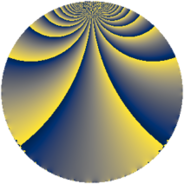# Properties

 Label 140.2.oLevel $140$ Weight $2$ Character orbit 140.o Rep. character $\chi_{140}(31,\cdot)$ Character field $\Q(\zeta_{6})$ Dimension $32$ Newform subspaces $1$ Sturm bound $48$ Trace bound $0$

# Related objects

## Defining parameters

 Level: $$N$$ $$=$$ $$140 = 2^{2} \cdot 5 \cdot 7$$ Weight: $$k$$ $$=$$ $$2$$ Character orbit: $$[\chi]$$ $$=$$ 140.o (of order $$6$$ and degree $$2$$) Character conductor: $$\operatorname{cond}(\chi)$$ $$=$$ $$28$$ Character field: $$\Q(\zeta_{6})$$ Newform subspaces: $$1$$ Sturm bound: $$48$$ Trace bound: $$0$$

## Dimensions

The following table gives the dimensions of various subspaces of $$M_{2}(140, [\chi])$$.

Total New Old
Modular forms 56 32 24
Cusp forms 40 32 8
Eisenstein series 16 0 16

## Trace form

 $$32q + 2q^{2} - 2q^{4} - 4q^{8} - 16q^{9} + O(q^{10})$$ $$32q + 2q^{2} - 2q^{4} - 4q^{8} - 16q^{9} - 30q^{12} + 2q^{14} - 14q^{16} - 12q^{21} - 8q^{22} + 36q^{24} + 16q^{25} + 30q^{26} + 2q^{28} - 40q^{29} + 2q^{32} + 60q^{36} + 8q^{37} - 60q^{38} - 62q^{42} - 18q^{44} + 12q^{45} + 2q^{46} - 16q^{49} + 4q^{50} - 36q^{52} - 8q^{53} + 12q^{54} - 4q^{56} + 48q^{57} + 2q^{58} + 14q^{60} + 24q^{61} + 4q^{64} + 4q^{65} + 24q^{66} + 60q^{68} - 4q^{70} + 4q^{72} - 72q^{73} + 38q^{74} - 40q^{77} + 120q^{78} - 36q^{81} + 42q^{82} - 20q^{84} + 28q^{86} + 4q^{88} - 60q^{89} - 4q^{92} - 8q^{93} + 18q^{94} - 60q^{96} + 78q^{98} + O(q^{100})$$

## Decomposition of $$S_{2}^{\mathrm{new}}(140, [\chi])$$ into newform subspaces

Label Dim. $$A$$ Field CM Traces $q$-expansion
$$a_2$$ $$a_3$$ $$a_5$$ $$a_7$$
140.2.o.a $$32$$ $$1.118$$ None $$2$$ $$0$$ $$0$$ $$0$$

## Decomposition of $$S_{2}^{\mathrm{old}}(140, [\chi])$$ into lower level spaces

$$S_{2}^{\mathrm{old}}(140, [\chi]) \cong$$ $$S_{2}^{\mathrm{new}}(28, [\chi])$$$$^{\oplus 2}$$